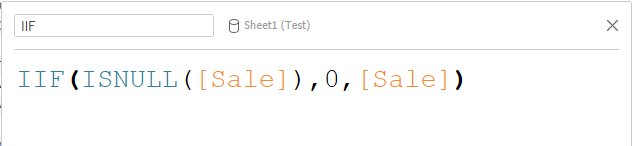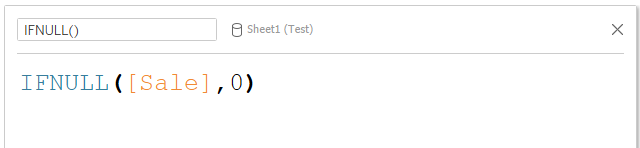top of page
Search
• Bernard K

# Dealing with NULL values in Tableau

Updated: May 17, 2021NULL values are blanks that often appear in data sets, they could be intentionally blanks (representing zero) or simply incomplete data. NULL values can affect results of our computations differently depending on how you use them.

In this article, am going to share different functions you can use to handle NULL values in Tableau.

### Use ZN() function

ZN() function simply assigns the value zero to the null values. Useful when you need to convert NULL values to zeros in your computation. Think of a scenario where data on Sales has been captured as blank when in the actual sense it was a zero sale. Such data issues can be addressed using this function.

Example(In the above example, using ZN() function have assigned the value zero to NULL values)

### Use ISNULL() function

You can also use ISNULL() function to achieve the same results. Basically ISNULL() function tests a numerical column returning ‘TRUE’ if the expression doesn’t contain a valid data (null).

Such function could also be used in a calculation as follows.(This function simply assigns zero for cases where the function ISNULL() is TRUE)

The above calculation can also be re-written using IIF (if and only if) function as follows.### Use IFNULL() function

This function returns the current value <expr 1> if it is not null, otherwise it returns your preferred value <expr 2>

This function can also be used to assign the value zero to null values as shown in the calculation below.Looking at the results of the above four functions are the same.The four functions provide ways in which we can deal with NULL values by assigning them value zero. Other ways of dealing with null values include, hiding or excluding them in your analysis.